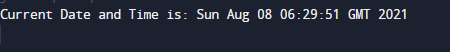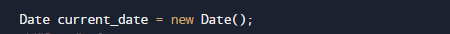# Java Program to Get Current Date/Time

In this tutorial you will learn about the Java Program to Get Current Date/Time and its application with practical example.

In this tutorial, we will learn to create a Java program that will Print Current Date/Time using Java programming.

## Prerequisites

Before starting with this tutorial we assume that you are best aware of the following Java programming topics:

• Java Operators.
• Basic Input and Output
• Class and Object.
• Basic Java programming.
• Array.
• User Define Functions.
• in-built library functions.

# Getting Current Date and Time in Java.

There are so many ways to get current date and time in java. Java provides so many classes that can be used to get current date and time in java.Let’s have a look at some of them.

1. java.time.Clock class.
2. java.time.LocalDate class.
3. java.time.format.DateTimeFormatter class.
4. java.util.Date class.
5. java.util.Calendar class.

## Java Program to Get Current Date/Time

In this program we will create a program to find Current Date/Time. We would first declared and initialized the required variables.Later we add all the elements and find Current Date/Time.let’s have a look at the code

## OutputIn the above program, the current date and time is stored in variable current_date using Date() method.

Here how the above program code works:

Here  we use class called Date class which represents the current date and time in Greenwich Mean Time. We can Display time in Indian Standard time also by  adding 5 hours and 30 minutes .This class comes under the import java.util.* package of Java.As you can see in our program we use in-built function to get Current Date and Time using Date() method which is define in import  java.util.*; package.

First we declare a variable current_date and with the Date() Method we get the current Date/time  of system. and simplyprint Current date and time of system by default this function gives value in GMT(Greenwich Mean Time) we can show the value in Indian Standard Time by adding +5.30 in GMT to get Indian time.

In this tutorial we have learn about the Java Program to Get Current Date/Time and its application with practical example. I hope you will like this tutorial.